# RS Aggarwal Solutions for Class 8 Chapter 3 - Square and Square Roots Exercise 3B

Students can refer and download the RS Aggarwal Solutions for the Exercise 3B of Class 8 Maths Chapter 3, Squares and Square Roots from the link available here. At BYJU’S, we have a set of expert faculties trying their best to provide exercise-wise solutions to students according to their level of understanding. This exercise can be used as a model of reference by the students to improve their conceptual knowledge and understand the different ways used to solve the problems. Exercise 3B of class 8 helps students to understand the concepts of perfect square properties as well as the product of two consecutive odd or consecutive even numbers. By practising the RS Aggarwal Solutions for class 8, students will be able to grasp the concepts perfectly. It also helps in boosting their confidence, which plays a crucial role in their examinations.

## Download PDF of RS Aggarwal Solutions for Class 8 Chapter 3 – Squares and Square Roots – Exercise 3B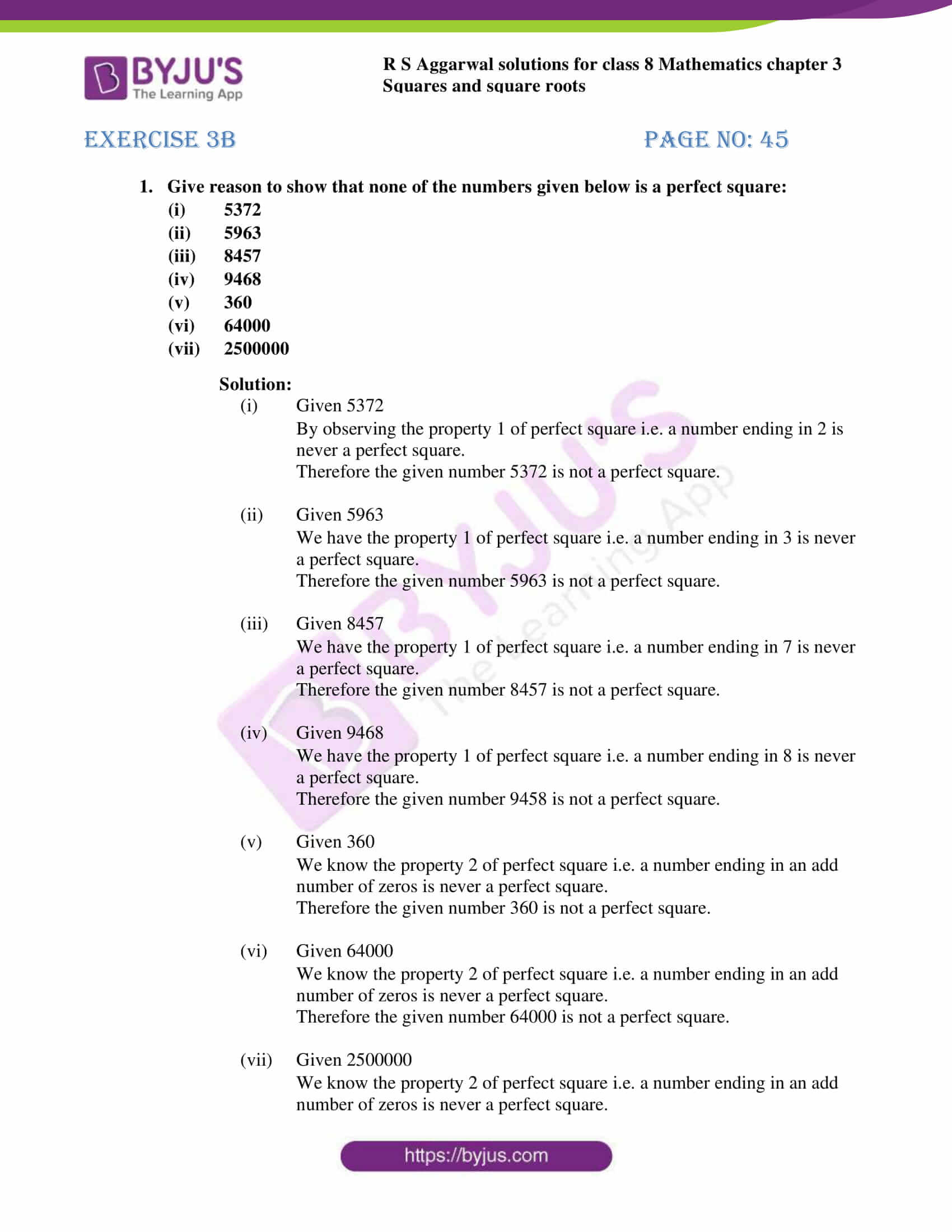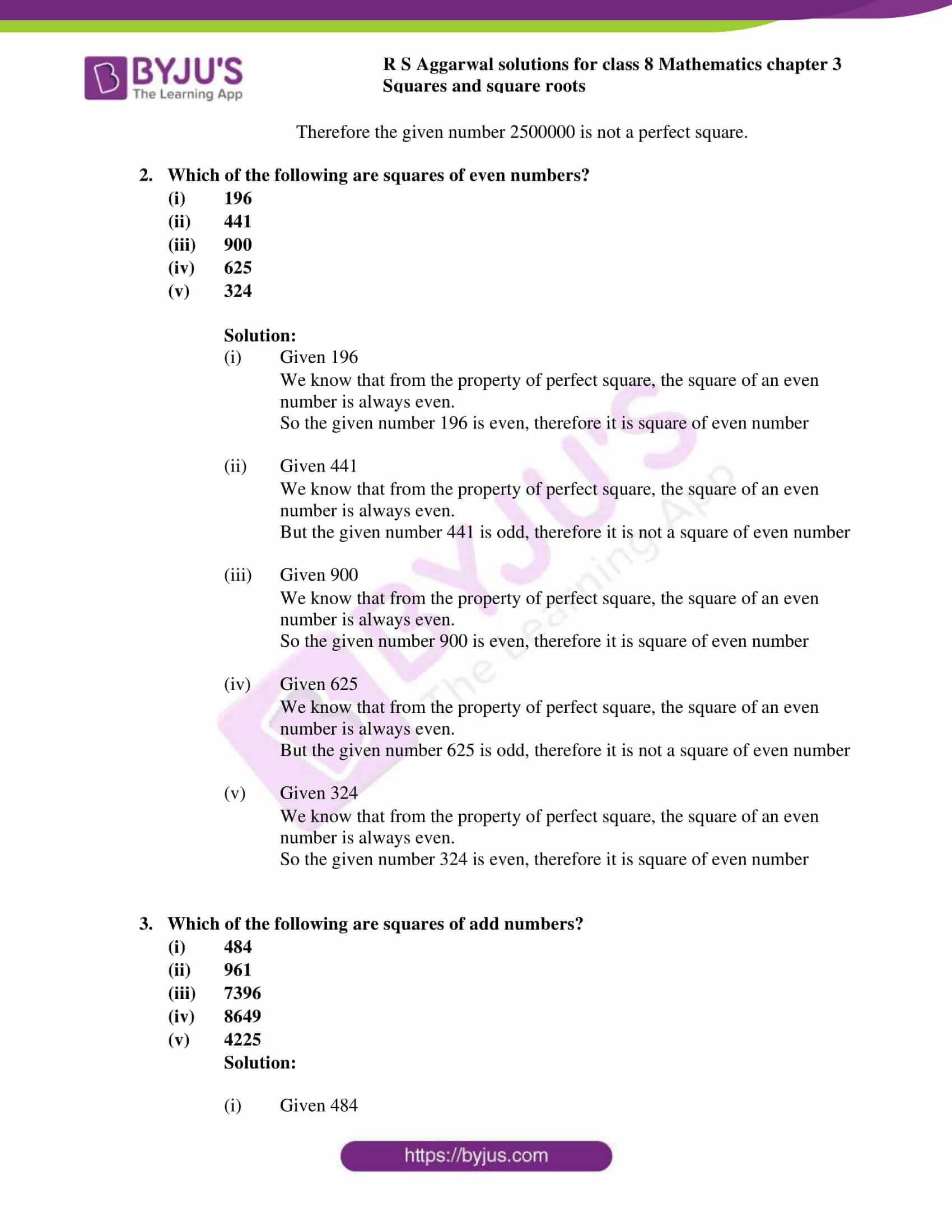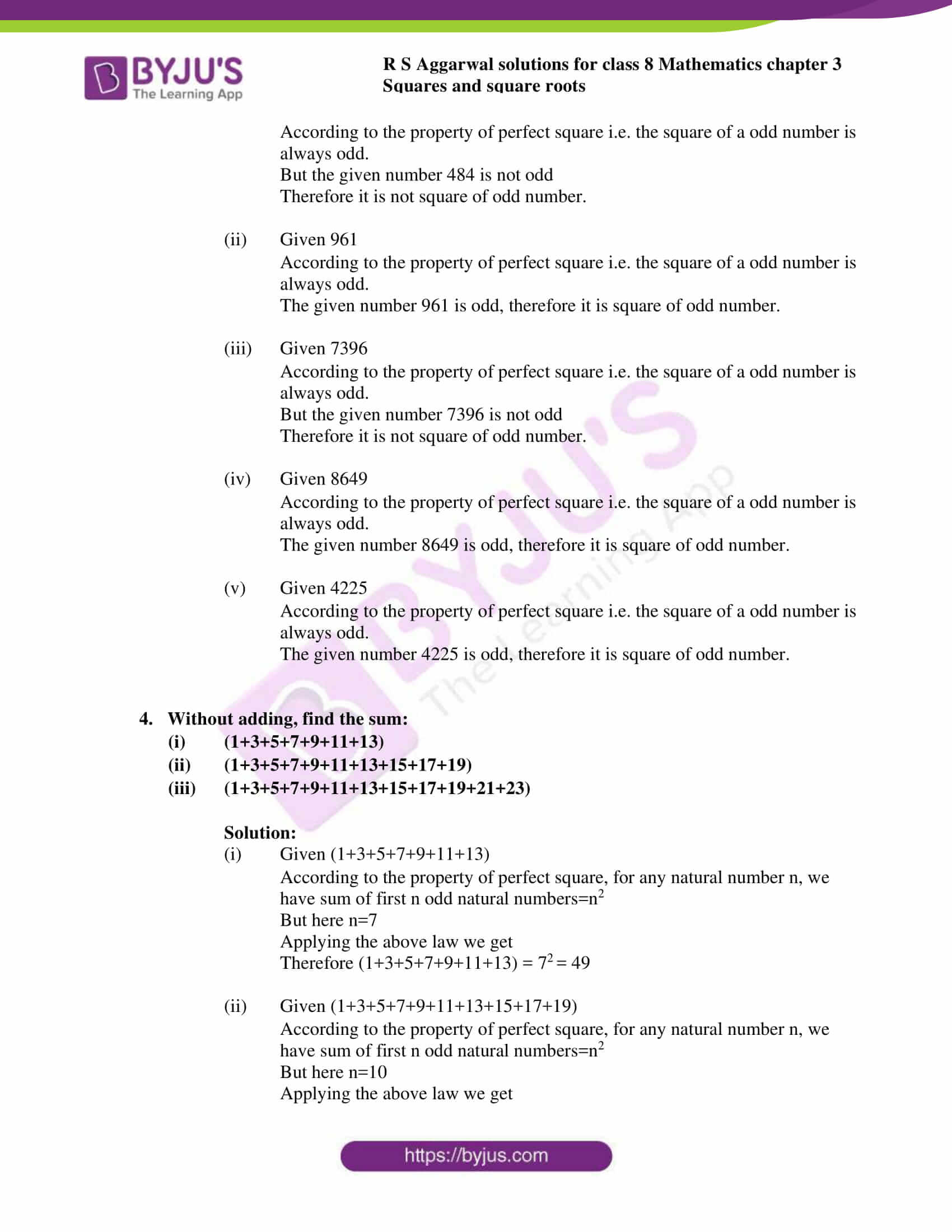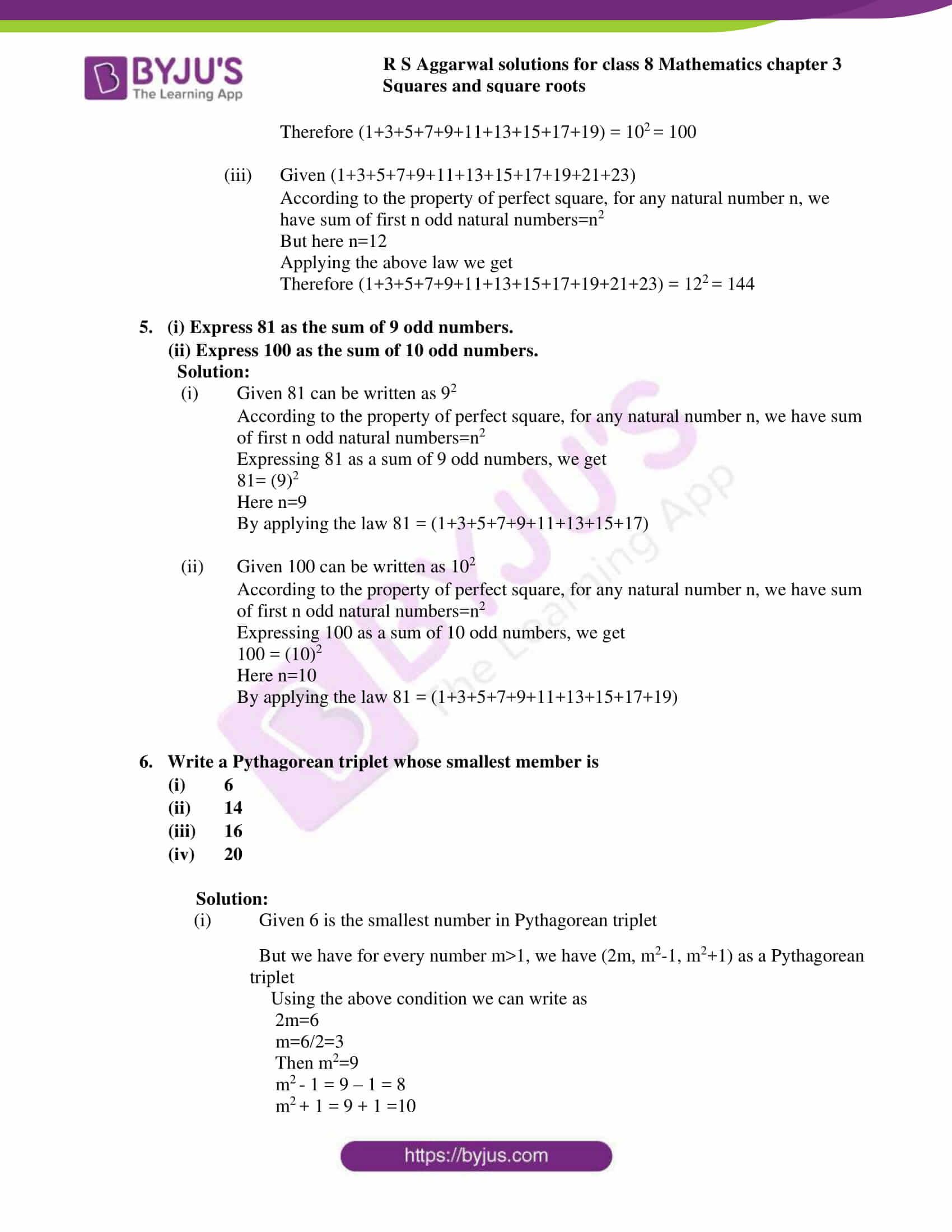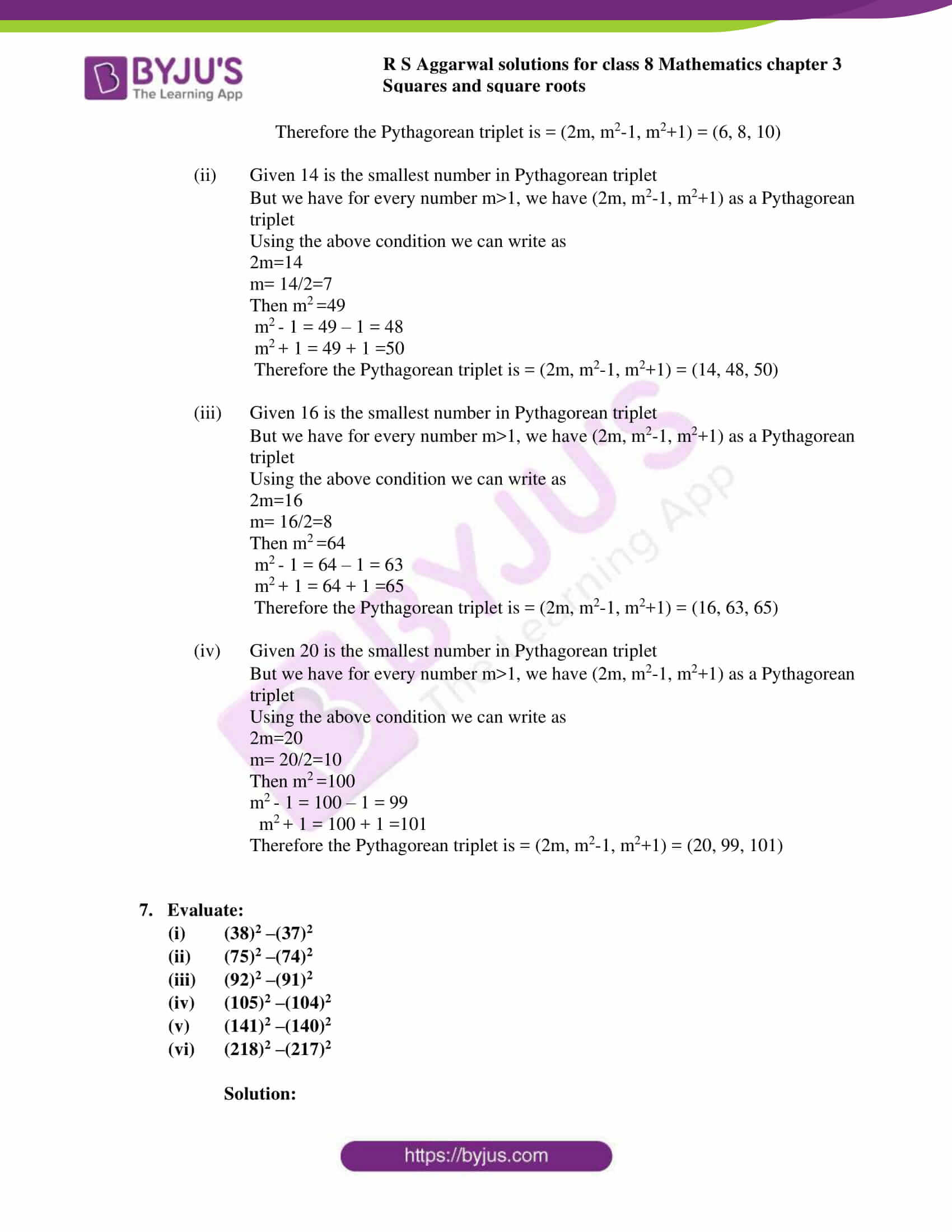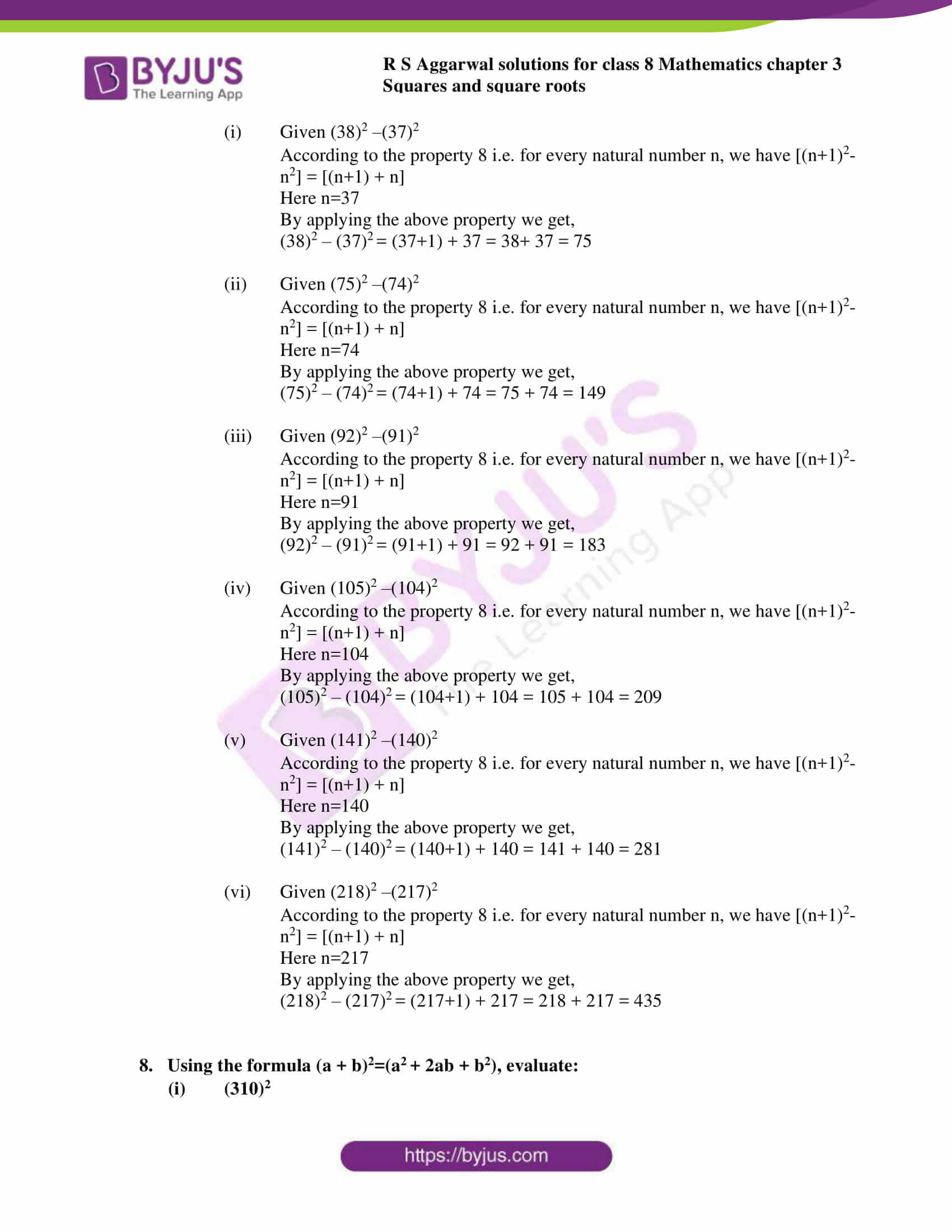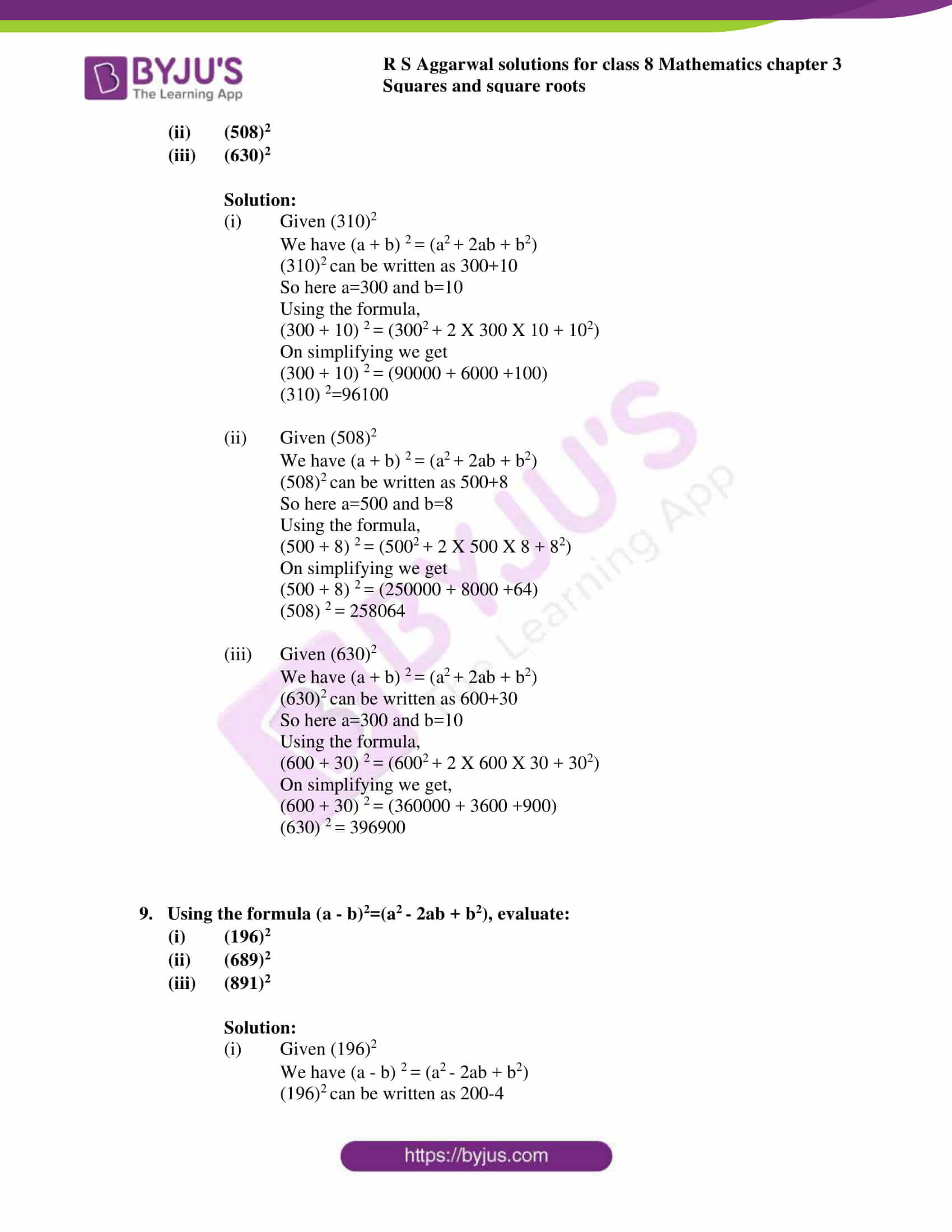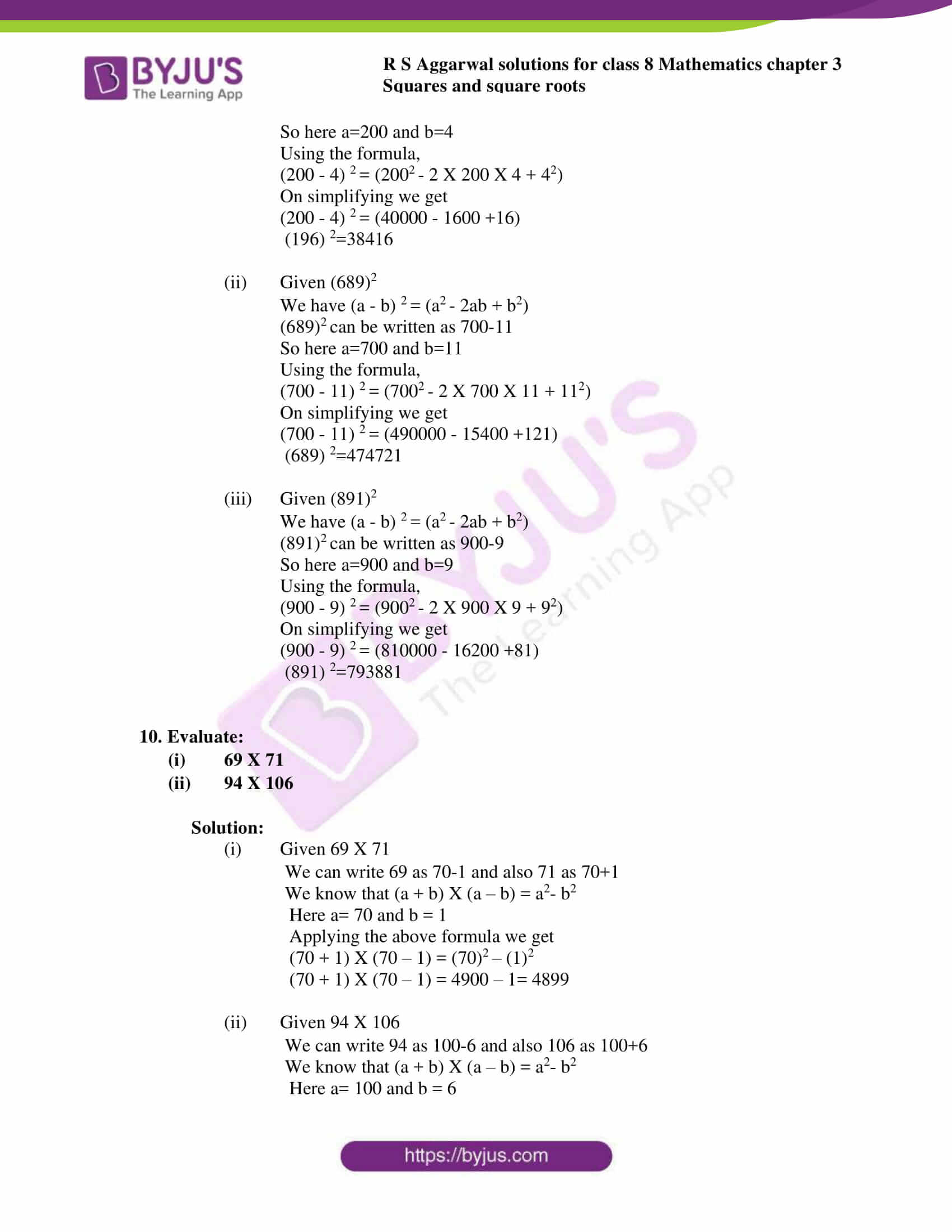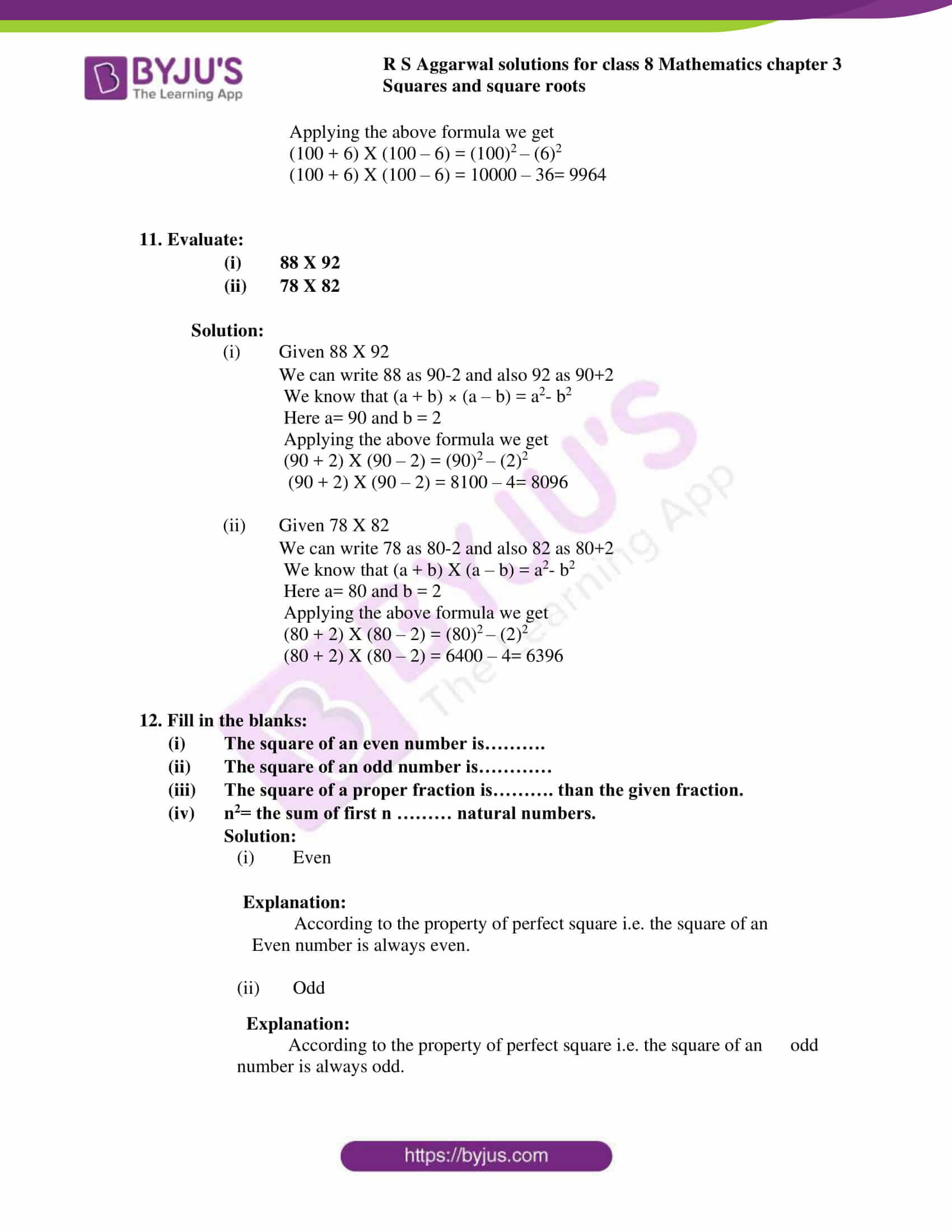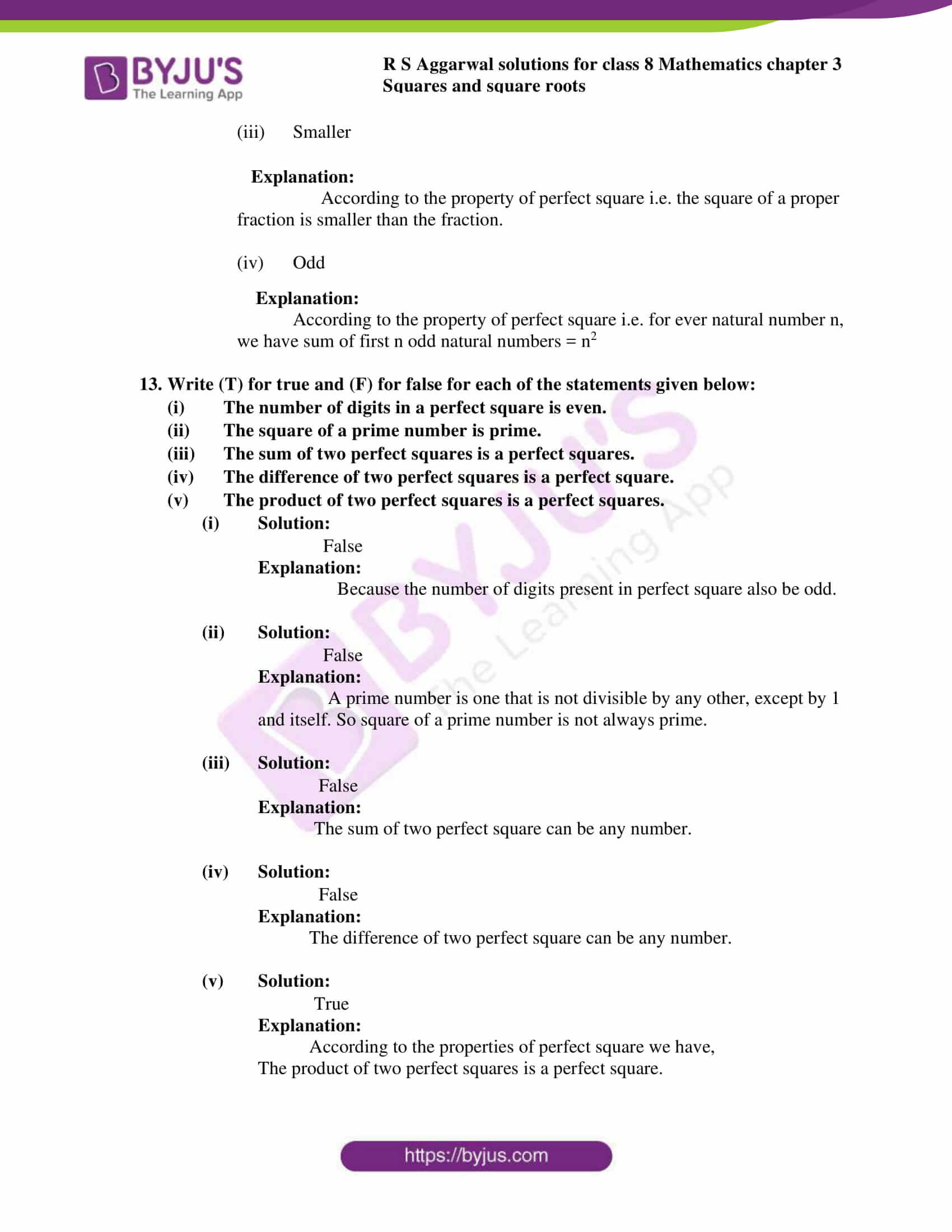### Access answers to Maths RS Aggarwal Solutions for Class 8 Chapter 3 – Squares and Square Roots Exercise 3B

Q1. Give reason to show that none of the numbers given below is a perfect square:

(i) 5372

(ii) 5963

(iii) 8457

(iv) 9468

(v) 360

(vi) 64000

(vii) 2500000

Solution:

1. Given 5372By observing the property 1 of perfect square i.e. a number ending in 2 is never a perfect square.Therefore the given number 5372 is not a perfect square.
2. Given 5963We have the property 1 of perfect square i.e. a number ending in 3 is never a perfect square.Therefore the given number 5963 is not a perfect square.
3. Given 8457We have the property 1 of perfect square i.e. a number ending in 7 is never a perfect square.Therefore the given number 8457 is not a perfect square.
4. Given 9468We have the property 1 of perfect square i.e. a number ending in 8 is never a perfect square.Therefore the given number 9458 is not a perfect square.
5. Given 360We know the property 2 of perfect square i.e. a number ending in an add number of zeros is never a perfect square.Therefore the given number 360 is not a perfect square.
6. Given 64000We know the property 2 of perfect square i.e. a number ending in an add number of zeros is never a perfect square.Therefore the given number 64000 is not a perfect square.
7. Given 2500000We know the property 2 of perfect square i.e. a number ending in an add number of zeros is never a perfect square.Therefore the given number 2500000 is not a perfect square.

Q2. Which of the following are squares of even numbers?

(i) 196

(ii) 441

(iii) 900

(iv) 625

(v) 324

Solution:

1. Given 196We know that from the property of perfect square, the square of an even number is always even.So the given number 196 is even, therefore it is square of even number
2. Given 441We know that from the property of perfect square, the square of an even number is always even.But the given number 441 is odd, therefore it is not a square of even number
3. Given 900We know that from the property of perfect square, the square of an even number is always even.So the given number 900 is even, therefore it is square of even number
4. Given 625We know that from the property of perfect square, the square of an even number is always even.But the given number 625 is odd, therefore it is not a square of even number
5. Given 324We know that from the property of perfect square, the square of an even number is always even.So the given number 324 is even, therefore it is square of even number

Q3. Which of the following are squares of add numbers?

(i) 484

(ii) 961

(iii) 7396

(iv) 8649

(v) 4225

Solution:

1. Given 484According to the property of perfect square i.e. the square of a odd number is always odd.But the given number 484 is not oddTherefore it is not square of odd number.
2. Given 961According to the property of perfect square i.e. the square of a odd number is always odd.The given number 961 is odd, therefore it is square of odd number.
3. Given 7396According to the property of perfect square i.e. the square of a odd number is always odd.But the given number 7396 is not oddTherefore it is not square of odd number.
4. Given 8649According to the property of perfect square i.e. the square of a odd number is always odd.The given number 8649 is odd, therefore it is square of odd number.
5. Given 4225According to the property of perfect square i.e. the square of a odd number is always odd.The given number 4225 is odd, therefore it is square of odd number.

Q4. Without adding, find the sum:

(i) (1+3+5+7+9+11+13)

(ii) (1+3+5+7+9+11+13+15+17+19)

(iii) (1+3+5+7+9+11+13+15+17+19+21+23)

Solution:

1. Given (1+3+5+7+9+11+13)According to the property of perfect square, for any natural number n, we have sum of first n odd natural numbers=n2But here n=7Applying the above law we get

Therefore (1+3+5+7+9+11+13) = 72 = 49

2. Given (1+3+5+7+9+11+13+15+17+19)According to the property of perfect square, for any natural number n, we have sum of first n odd natural numbers=n2But here n=10Applying the above law we get

Therefore (1+3+5+7+9+11+13+15+17+19) = 102 = 100

3. Given (1+3+5+7+9+11+13+15+17+19+21+23)According to the property of perfect square, for any natural number n, we have sum of first n odd natural numbers=n2But here n=12Applying the above law we get

Therefore (1+3+5+7+9+11+13+15+17+19+21+23) = 122 = 144

Q5.

(i) Express 81 as the sum of 9 odd numbers.

(ii) Express 100 as the sum of 10 odd numbers.

Solution:

1. Given 81 can be written as 92According to the property of perfect square, for any natural number n, we have sum of first n odd natural numbers=n2Expressing 81 as a sum of 9 odd numbers, we get81= (9)2

Here n=9

By applying the law 81 = (1+3+5+7+9+11+13+15+17)

2. Given 100 can be written as 102According to the property of perfect square, for any natural number n, we have sum of first n odd natural numbers=n2Expressing 100 as a sum of 10 odd numbers, we get100 = (10)2

Here n=10

By applying the law 81 = (1+3+5+7+9+11+13+15+17+19)

Q6. Write a Pythagorean triplet whose smallest member is

(i) 6

(ii) 14

(iii) 16

(iv) 20

Solution:

1. Given 6 is the smallest number in Pythagorean tripletBut we have for every number m>1, we have (2m, m2-1, m2+1) as a Pythagorean tripletUsing the above condition we can write as2m=6

m=6/2=3

Then m2=9

m2 – 1 = 9 – 1 = 8

m2 + 1 = 9 + 1 =10

Therefore the Pythagorean triplet is = (2m, m2-1, m2+1) = (6, 8, 10)

2. Given 14 is the smallest number in Pythagorean tripletBut we have for every number m>1, we have (2m, m2-1, m2+1) as a Pythagorean tripletUsing the above condition we can write as2m=14

m= 14/2=7

Then m2 =49

m2 – 1 = 49 – 1 = 48

m2 + 1 = 49 + 1 =50

Therefore the Pythagorean triplet is = (2m, m2-1, m2+1) = (14, 48, 50)

3. Given 16 is the smallest number in Pythagorean tripletBut we have for every number m>1, we have (2m, m2-1, m2+1) as a Pythagorean tripletUsing the above condition we can write as2m=16

m= 16/2=8

Then m2 =64

m2 – 1 = 64 – 1 = 63

m2 + 1 = 64 + 1 =65

Therefore the Pythagorean triplet is = (2m, m2-1, m2+1) = (16, 63, 65)

4. Given 20 is the smallest number in Pythagorean tripletBut we have for every number m>1, we have (2m, m2-1, m2+1) as a Pythagorean tripletUsing the above condition we can write as2m=20

m= 20/2=10

Then m2 =100

m2 – 1 = 100 – 1 = 99

m2 + 1 = 100 + 1 =101

Therefore the Pythagorean triplet is = (2m, m2-1, m2+1) = (20, 99, 101)

Q7. Evaluate:

(i) (38)2 –(37)2

(ii) (75)2 –(74)2

(iii) (92)2 –(91)2

(iv) (105)2 –(104)2

(v) (141)2 –(140)2

(vi) (218)2 –(217)2

Solution:

1. Given (38)2 –(37)2According to the property 8 i.e. for every natural number n, we have [(n+1)2-n2] = [(n+1) + n]Here n=37By applying the above property we get,

(38)2 – (37)2 = (37+1) + 37 = 38+ 37 = 75

2. Given (75)2 –(74)2According to the property 8 i.e. for every natural number n, we have [(n+1)2-n2] = [(n+1) + n]Here n=74By applying the above property we get,

(75)2 – (74)2 = (74+1) + 74 = 75 + 74 = 149

3. Given (92)2 –(91)2According to the property 8 i.e. for every natural number n, we have [(n+1)2-n2] = [(n+1) + n]Here n=91By applying the above property we get,

(92)2 – (91)2 = (91+1) + 91 = 92 + 91 = 183

4. Given (105)2 –(104)2According to the property 8 i.e. for every natural number n, we have [(n+1)2-n2] = [(n+1) + n]Here n=104By applying the above property we get,

(105)2 – (104)2 = (104+1) + 104 = 105 + 104 = 209

5. Given (141)2 –(140)2According to the property 8 i.e. for every natural number n, we have [(n+1)2-n2] = [(n+1) + n]Here n=140By applying the above property we get,

(141)2 – (140)2 = (140+1) + 140 = 141 + 140 = 281

6. Given (218)2 –(217)2According to the property 8 i.e. for every natural number n, we have [(n+1)2-n2] = [(n+1) + n]Here n=217By applying the above property we get,

= – (217)2 = (217+1) + 217 = 218 + 217 = 435

Q8. Using the formula (a + b)2=(a2 + 2ab + b2), evaluate:

(i) (310)2

(ii) (508)2

(iii) (630)2

Solution:

1. Given (310)2We have (a + b) 2 = (a2 + 2ab + b2)(310)2 can be written as 300+10So here a=300 and b=10

Using the formula,

(300 + 10) 2 = (3002 + 2 X 300 X 10 + 102)

On simplifying we get

(300 + 10) 2 = (90000 + 6000 +100)

(310) 2=96100

2. Given (508)2We have (a + b) 2 = (a2 + 2ab + b2)(508)2 can be written as 500+8So here a=500 and b=8

Using the formula,

(500 + 8) 2 = (5002 + 2 X 500 X 8 + 82)

On simplifying we get

(500 + 8) 2 = (250000 + 8000 +64)

(508) 2 = 258064

3. Given (630)2We have (a + b) 2 = (a2 + 2ab + b2)(630)2 can be written as 600+30So here a=300 and b=10

Using the formula,

(600 + 30) 2 = (6002 + 2 X 600 X 30 + 302)

On simplifying we get,

(600 + 30) 2 = (360000 + 3600 +900)

(630) 2 = 396900

Q9. Using the formula (a – b)2=(a2 – 2ab + b2), evaluate:

(i) (196)2

(ii) (689) 2

(iii) (891) 2

Solution:

1. Given (196)2We have (a – b) 2 = (a2 – 2ab + b2)(196)2 can be written as 200-4So here a=200 and b=4

Using the formula,

(200 – 4) 2 = (2002 – 2 X 200 X 4 + 42)

On simplifying we get

(200 – 4) 2 = (40000 – 1600 +16)

(196) 2=38416

2. Given (689)2We have (a – b) 2 = (a2 – 2ab + b2)(689)2 can be written as 700-11So here a=700 and b=11

Using the formula,

(700 – 11) 2 = (7002 – 2 X 700 X 11 + 112)

On simplifying we get

(700 – 11) 2 = (490000 – 15400 +121)

(689) 2=474721

3. Given (891)2We have (a – b) 2 = (a2 – 2ab + b2)(891)2 can be written as 900-9So here a=900 and b=9

Using the formula,

(900 – 9) 2 = (9002 – 2 X 900 X 9 + 92)

On simplifying we get

(900 – 9) 2 = (810000 – 16200 +81)

(891) 2=793881

Q10. Evaluate:

(i) 69 X 71

(ii) 94 X 106

Solution:

1. Given 69 X 71We can write 69 as 70-1 and also 71 as 70+1We know that (a + b) X (a – b) = a2– b2Here a= 70 and b = 1

Applying the above formula we get

(70 + 1) X (70 – 1) = (70)2 – (1)2

(70 + 1) X (70 – 1) = 4900 – 1= 4899

2. Given 94 X 106We can write 94 as 100-6 and also 106 as 100+6We know that (a + b) X (a – b) = a2– b2Here a= 100 and b = 6

Applying the above formula we get

(100 + 6) X (100 – 6) = (100)2 – (6)2

(100 + 6) X (100 – 6) = 10000 – 36= 9964

Q11. Evaluate:

(i) 88 X 92

(ii) 78 X 82

Solution:

1. Given 88 X 92We can write 88 as 90-2 and also 92 as 90+2We know that (a + b) × (a – b) = a2– b2Here a= 90 and b = 2

Applying the above formula we get

(90 + 2) X (90 – 2) = (90)2 – (2)2

(90 + 2) X (90 – 2) = 8100 – 4= 8096

2. Given 78 X 82We can write 78 as 80-2 and also 82 as 80+2We know that (a + b) X (a – b) = a2– b2Here a= 80 and b = 2

Applying the above formula we get

(80 + 2) X (80 – 2) = (80)2 – (2)2

(80 + 2) X (80 – 2) = 6400 – 4= 6396

Q12. Fill in the blanks:

(i) The square of an even number is……….

(ii) The square of an odd number is…………

(iii) The square of a proper fraction is………. than the given fraction.

(iv) n2= the sum of first n ……… natural numbers.

Solution:

1. Even Explanation: According to the property of perfect square i.e. the square of anEven number is always even.
2. Odd Explanation: According to the property of perfect square i.e. the square of an odd number is always odd.
3. SmallerExplanation: According to the property of perfect square i.e. the square of a proper fraction is smaller than the fraction.
4. OddExplanation: According to the property of perfect square i.e. for ever natural number n, we have sum of first n odd natural numbers = n2

Q13. Write (T) for true and (F) for false for each of the statements given below:

(i) The number of digits in a perfect square is even.

(ii) The square of a prime number is prime.

(iii) The sum of two perfect squares is a perfect squares.

(iv) The difference of two perfect squares is a perfect square.

(v) The product of two perfect squares is a perfect squares.

1. Solution:FalseExplanation:Because the number of digits present in perfect square also be odd.
2. Solution: FalseExplanation: A prime number is one that is not divisible by any other, except by 1 and itself. So square of a prime number is not always prime.
3. Solution: FalseExplanation: The sum of two perfect square can be any number.
4. Solution: FalseExplanation: The difference of two perfect square can be any number.
5. Solution: TrueExplanation: According to the properties of perfect square we have,

The product of two perfect squares is a perfect square.

### Access other exercises of RS Aggarwal Solutions for Class 8 Chapter 3 – Squares and Square Roots

Exercise 3A Solutions 6 questions

Exercise 3C Solutions 8 questions

Exercise 3D Solutions 18 questions

Exercise 3E Solutions 19 questions

Exercise 3F Solutions 12 questions

Exercise 3G Solutions 10 questions

Exercise 3H Solutions 19 questions

## RS Aggarwal Solutions for Class 8 Maths Chapter 3 – Squares and Square Roots Exercise 3B

Exercise 3B of RS Aggarwal Solutions for Chapter 3, Squares and Square Roots deals with the basic concepts related to properties of a perfect square. We can say that this exercise mainly deals with the properties of perfect squares such as Pythagorean Triplets, the sum of first ‘n’ odd natural numbers, etc. that the students have learned in this chapter. This exercise contains 13 main questions and all questions are based on properties of perfect squares. Some of the topics focused prior to exercise 3B include the following.

• Properties of a perfect square
• Pythagorean Triplets
• Product of two consecutive odd numbers
• Product of two consecutive even numbers